# Iterated Powers Fractal

Iterating powers of complex numbers can produce cycles. Colormaps of the lengths of these cycles produce fractal images.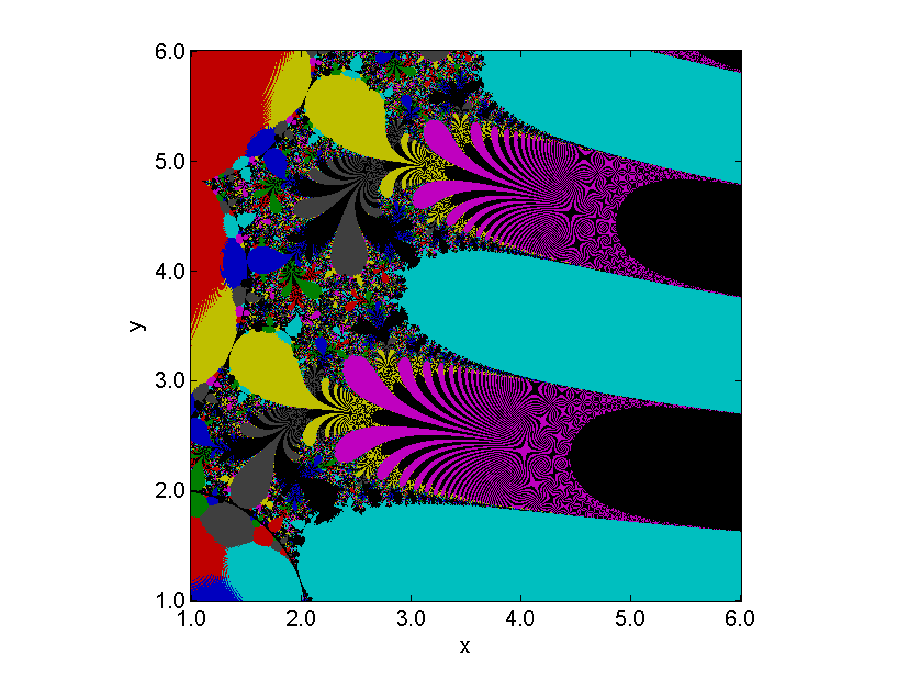### Contents

#### UWO Poster

In my blog a month ago I mentioned a poster produced at the University of Western Ontario about the Lambert W function. And in my blog two weeks ago about the tower of powers $$z^{z^{z^z}}$$ I said that if the base $z$ is less that $\mbox{e}^{-\mbox{e}}$, which is about 0.066, then the iteration evaluating the tower settles into a stable two-cycle. Both of these posts lead to today's blog where $z$ moves in the complex plane.

#### The Iteration

The base of the iteration is a real or complex number $z$ that stays fixed throughout the iteration. Start with $y_0 = 1$ and simply iterate $$y_{k+1} = z^{y_k}$$ If $z$ is too large, then $y_k$ will quickly blow up. As we saw in my last blog, this happens for real $z$ larger than $\mbox{e}^{1/\mbox{e}}$, which is about $1.444$. For some $z$, the iterates $y_k$ approach a fixed point. An example is $z = \sqrt{2}$. The $y_k$ converge to $2$. We regard fixed points as cycles of length 1.

#### An Example

Here is an example of a complex $z$ that produces a cycle of length 7, $z = 2.5+4i$.
   format short
z = 2.5+4i
y = 1
for k = 1:7
y = z^y;
disp(y)
end

z =
2.5000 + 4.0000i
y =
1
2.5000 + 4.0000i
-0.6503 + 0.5363i
0.2087 + 0.0366i
1.2845 + 0.3528i
-1.4011 + 4.9361i
7.6883e-04 - 3.4362e-05i
1.0012 + 0.0007i

After 7 steps, we're not quite back at y = 1, but we're pretty close. Repeating the iteration a few dozen times brings us to a stable cycle of length 7 near this initial transient. Here is a picture.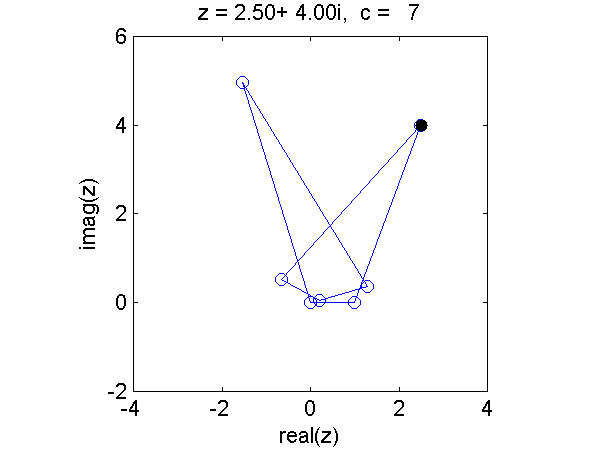#### Cycle Length

Finding cycle length involves saving the iterates on a stack and comparing each new iterate with the previous ones. The internal parameters of the function are the tolerance in the comparison, the stack size, and the maximum number of iterations. The cycle length is declared to be infinite if either the iterates go off to infinity or no match is found before the iteration limit is reached. Here is the function.
   type cycle

function c = cycle(z)
% CYCLE  Cycle length of iterated power
% c = cycle(z)
tol = 1.e-4;   % Tolerance
N = 40;        % Height of stack
M = 2000;      % Maximum number of iterations
S = zeros(N,1);   % Stack
S(:) = NaN;
y = 1;
k = 0;
c = Inf;
while k < M
y = z^y;
k = k+1;
if isinf(y)
break
elseif any(abs(y-S) < tol)
c = min(find(abs(y-S) < tol));
break
end
S(2:N) = S(1:N-1);
S(1) = y;
end
end


Let's test the function on a few cases where we already know the answer.
   cycle(sqrt(2))  % Should be 1
cycle(1.5)      % Should be Inf
cycle(.05)      % Should be 2
cycle(2.5+4i)   % Should be 7

ans =
1
ans =
Inf
ans =
2
ans =
7


#### Colormaps

Good colormaps are an important aspect of generating good fractal images. I am using the colormaps from the Mandelbrot chapter of Experiments with MATLAB. These take small chunks of the colormaps from MATLAB itself and repeat them several times. The maps are called jets, hots, and cmyk and are included in the mandelbrot.m code. Here is one of them.
   type jets

function cmap = jets(m)
c = jet(16);
e = ones(m/16,1);
cmap = kron(e,c);
end



#### Generate an Image

To generate a fractal image, put a grid over a region in the complex region, compute the cycle length for each grid point, and then use the MATLAB image function and one of the colormaps to produce a pseudocolor image. (This is an embarrassingly parallel task and has for many years been used to demonstrate parallel computers. Load balancing is a challenge. But I digress ...)

#### First Image

The image at the top of this post shows a large chunk of the complex plane. With the cmyk colormap, the infinite cycle lengths come out black. I can see that with a spy plot on my machine where I have saved the data.
   load view1
spy(isinf(u))
set(gca,'ydir','n')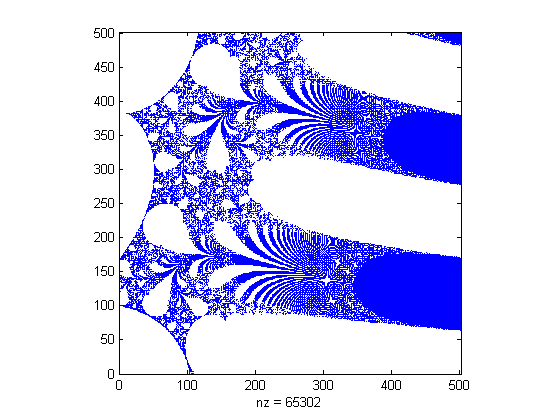While I am it, let's check the density of Inf's.
   nnz(isinf(u))/prod(size(u))

ans =
0.2602

Over a quarter of the grid points have infinite cycle lengths.

#### Second Image

Zoom in on a smaller region in the plane, and use the jets colormap with its columns flipped. This is typical of the structure we see everywhere in this fractal, at all magnification levels. They are certainly not as rich as the Mandelbrot set, but I still them interesting.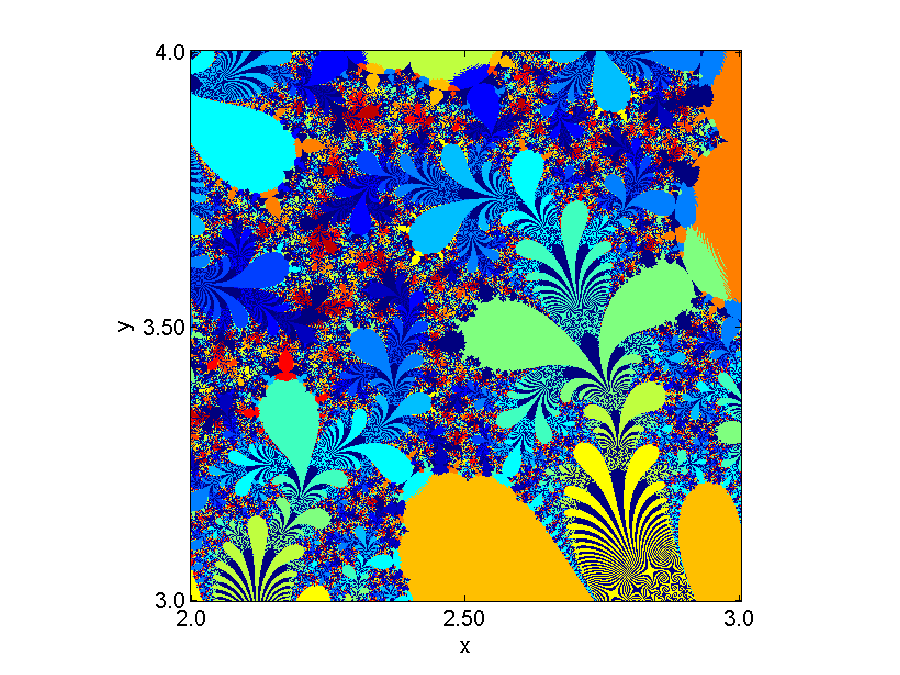#### Third Image

Zoom in farther, and use the hots colormap. The large brown region in the northwest corner is not infinite cycle length. It happens to be cycle == 16.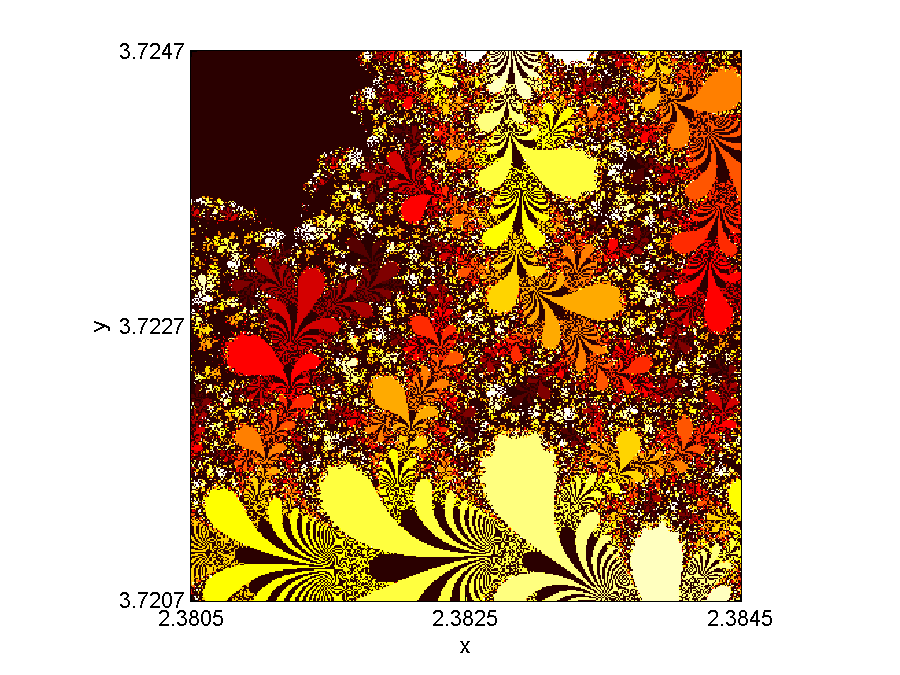Here is the code that generates this image. It takes about 5 minutes to run on my laptop, which is a couple of years old.
   type view3

x0 = 2.3825;
y0 = 3.7227;
d = 1.e-5;
nhalf = 200;
v = d*(-nhalf:nhalf);
[x,y] = meshgrid(x0+v,y0+v);
z = x+i*y;
u = zeros(size(z));

n = size(z,1)
tic
for k = 1:n
for j = 1:n
u(k,j) = cycle(z(k,j));
end
k
end
toc

image(u)
axis square
colormap(hots(128))
set(gca,'ydir','n')
set(gca,'xtick',[1,n/2,n])
set(gca,'ytick',[1,n/2,n])
set(gca,'xticklabel',{min(x(:)),num2str(x0),max(x(:))})
set(gca,'yticklabel',{min(y(:)),num2str(y0),max(y(:))})
xlabel('x')
ylabel('y')



#### Reference

The Lambert W Function, Poster, Ontario Research Centre for Computer Algebra, http://www.orcca.on.ca/LambertW/

Published with MATLAB® R2013b
|

### 댓글

댓글을 남기려면 링크 를 클릭하여 MathWorks 계정에 로그인하거나 계정을 새로 만드십시오.